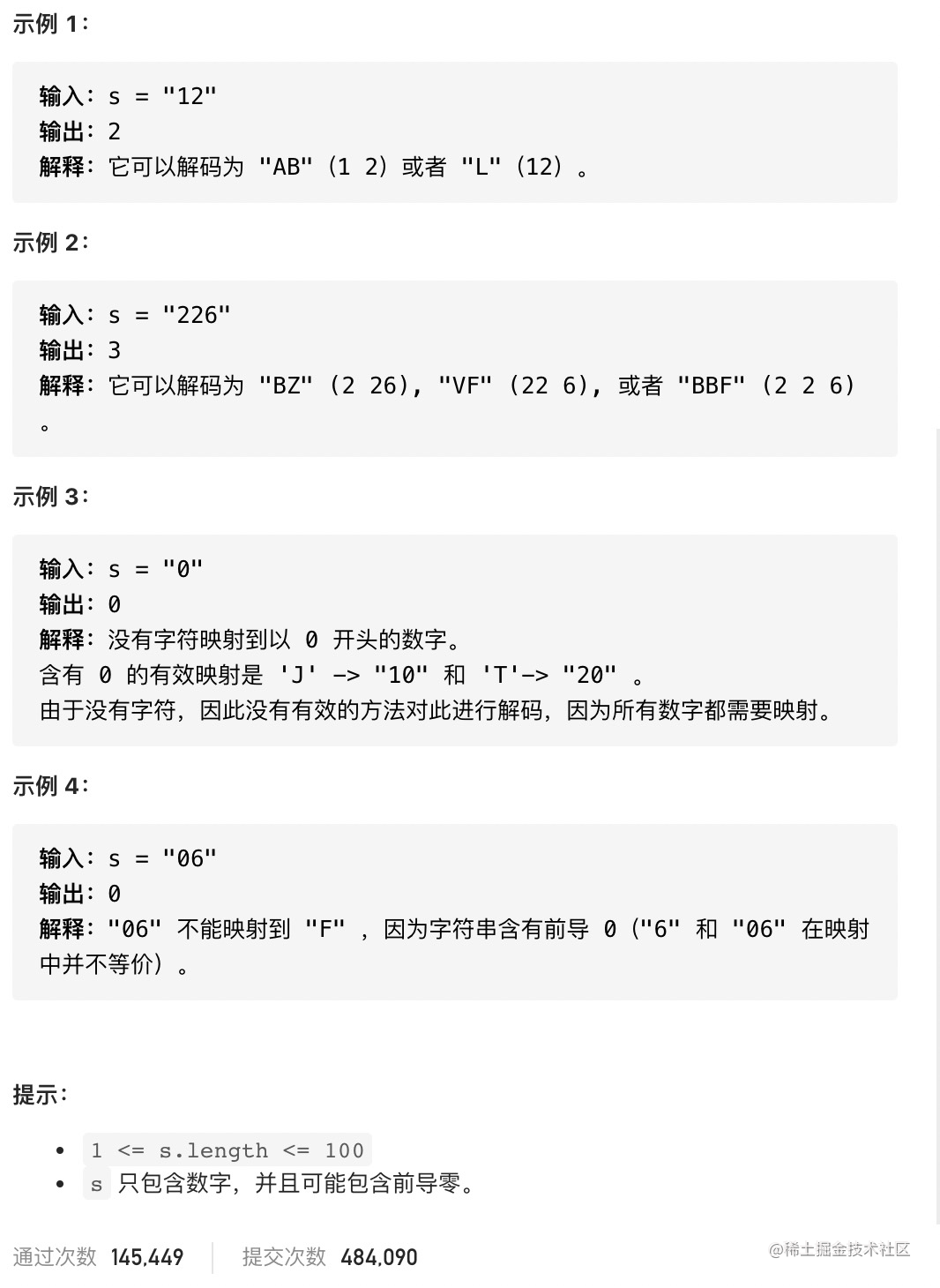# Leetcode 91. 解码方法 --javascript dp

### 描述

``````'A' -> 1
'B' -> 2
...
'Z' -> 26

``````"AAJF" ，将消息分组为 (1 1 10 6)
"KJF" ，将消息分组为 (11 10 6)## 题解

### 动态规划（Dynamic Programming, DP）

• 动态规划只能`应用于有最优 子结构`的问题。最优子结构的意思是`局部最优解能决定全局最优解`（对有些问题这个要求并不能完全满足，故有时需要引入一定的近似）。

• 简单地说，`问题能够分解成子问题来解决`

• 通俗一点来讲，动态规划和其它遍历算法（如深/广度优先搜索）都是将`原问题拆成多个子问题然后求解`，他们之间最本质的区别是，`动态规划保存子问题的解，避免重复计算`

• 解决动态规划问题的关键是找到`状态转移方程`，这样我们可以通`计算和储存子问题的解来求解最终问题`

• 同时，我们也可以对动态规划进行`空间压缩`，起到节省空间消耗的效果。

• 在一些情况下，动态规划可以看成是`带有状态记录（memoization）的优先搜索`

• `动态规划是自下而上的`，即先解决子问题，再解决父问题；

• 而用带有`状态记录的优先搜索``自上而下`的，即从父问题搜索到子问题，若重复搜索到同一个子问题则进行状态记录，防止重复计算。

• 如果题目需求的是最终状态，那么使用动态搜索比较方便；

• 如果题目需要输出所有的路径，那么使用带有状态记录的优先搜索会比较方便。

### 回到本题目

f_i = f_(i-1), 其中 s[i] !== '0'

f_i = f_(i-2), 其中 s[i-1] !== '0' 并且10 * s[i-1]+s[i] <= 26

## coding

``````/**
* @param {string} s
* @return {number}
*/
var numDecodings = function(s) {
let n = s.length;
if(n === 0) return 0;
if(!(s - '0')) return 0; // 前置0的干掉
if(n === 1) return 1;
const dp = new Array(n+1).fill(0);
dp = 1;
for(let i = 1; i <= n; i ++) {
if (s[i - 1] !== '0') {
dp[i] += dp[i - 1];
}
if (i > 1) {
if(s[i - 2] != '0' && ((s[i - 2] - '0') * 10 + (s[i - 1] - '0') <= 26)) {
dp[i] += dp[i - 2];
}

if((s[i-2] - '0' > 2 || s[i-2] === '') && s[i-1] === '0') return 0; // 中间不符合字母的数字干掉
}
}
return dp[n];

};